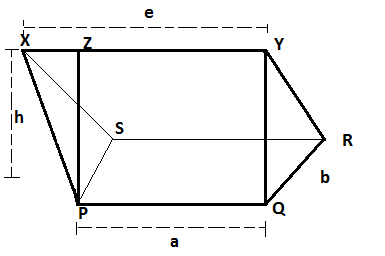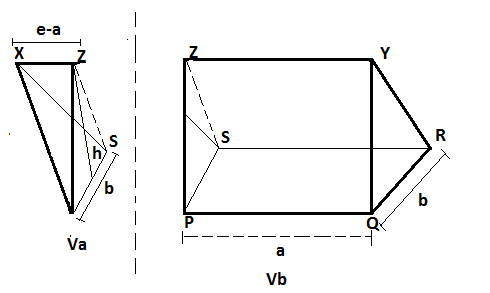# Find the volume of rectangular right wedge

A right wedge is a wedge with parallel side triangles. It have two side bases a and b, top edges e and height h. The task is to find the volume of the given rectangular right wedges.Examples:

Input: a = 2, b = 5, e = 5, h = 6
Output: Volume = 45.0

Input: a = 5, b = 4, e = 4, h = 6
Output: Volume = 56.0

## Recommended: Please try your approach on {IDE} first, before moving on to the solution.

Approach:Va is the volume of triangular pyramid i.e. Va = (1 / 3) * area of triangle * (e – a)
Area of triangle = (1 / 2) * b * h
i.e Va = ( 1 / 3 ) * (( 1 / 2 ) * ( b * h * (e – a)))
Vb is a volume of triangular prism i.e. Vb = area of cross section * length(side)
i.e. Vb = (1 / 2) * (b * h * a)

Total Volume = Va + Vb
= (1 / 3) * ((1 / 2) * (b * h * ( e – a ))) + (1 / 2) * (b * h * a)
= (1 / 6) * (b * h * (e – a)) + (1 / 2) * (b * h * a)
= ((b * h) * (e – a) + 3 * b * h * a) / 6
= (b * h * e – b * h * a + 3 * b * h * a) / 6
= (b * h * e + 2 * b * h * a) / 6
= (b * h / 6) * (2 * a + e)

Volume of the rectangular right wedge = (b * h / 6) * (2 * a + e) where a and b are the side bases, e is the top edge and h is the height of the rectangular right wedge.

Below is the implementation of the above approach:

## C++

 `// CPP program to find volume of rectangular right wedge  ` `#include   ` `using` `namespace` `std;  ` ` `  `// function to return volume ` `//of rectangular right wedge ` `double` `volumeRec(``double` `a,``double` `b,``double` `e,``double` `h) ` `{  ` `     `  `    ``return` `(((b * h )/ 6)*(2 * a + e)); ` `}  ` ` `  `// Driver code  ` `int` `main()  ` `{  ` `    ``double` `a = 2;  ` `    ``double` `b = 5;  ` `    ``double` `e = 5;  ` `    ``double` `h = 6;  ` `    ``printf``(``"Volume = %.1f"``,volumeRec(a, b, e, h)); ` `    ``return` `0;  ` `} ` ` `  `// This code contributed by nidhiva `

## Java

 `// Java implementation of the approach ` `class` `GFG { ` ` `  `    ``// Function to return the volume ` `    ``// of the rectangular right wedge ` `    ``static` `double` `volumeRec(``double` `a, ``double` `b, ``double` `e, ``double` `h) ` `    ``{ ` `        ``return` `(((b * h) / ``6``) * (``2` `* a + e)); ` `    ``} ` ` `  `    ``// Driver code ` `    ``public` `static` `void` `main(String[] args) ``throws` `java.lang.Exception ` `    ``{ ` `        ``double` `a = ``2``, b = ``5``, e = ``5``, h = ``6``; ` `        ``System.out.print(``"Volume = "` `+ volumeRec(a, b, e, h)); ` `    ``} ` `} `

## Python3

 `# Python3 implementation of the approach  ` ` `  `# Function to return the volume  ` `# of the rectangular right wedge  ` `def` `volumeRec(a, b, e, h) : ` `     `  `    ``return` `(((b ``*` `h) ``/` `6``) ``*` `(``2` `*` `a ``+` `e));  ` ` `  ` `  `# Driver code  ` `if` `__name__ ``=``=` `"__main__"` `: ` `     `  `    ``a ``=` `2``; b ``=` `5``; e ``=` `5``; h ``=` `6``;  ` `     `  `    ``print``(``"Volume = "``,volumeRec(a, b, e, h));  ` `     `  `# This code is contributed by AnkitRai01 `

## C#

 `// C# implementation of the approach ` `using` `System; ` ` `  `class` `GFG  ` `{ ` ` `  `    ``// Function to return the volume ` `    ``// of the rectangular right wedge ` `    ``static` `double` `volumeRec(``double` `a, ``double` `b,  ` `                            ``double` `e, ``double` `h) ` `    ``{ ` `        ``return` `(((b * h) / 6) * (2 * a + e)); ` `    ``} ` ` `  `    ``// Driver code ` `    ``public` `static` `void` `Main() ` `    ``{ ` `        ``double` `a = 2, b = 5, e = 5, h = 6; ` `        ``Console.WriteLine(``"Volume = "` `+ volumeRec(a, b, e, h)); ` `    ``} ` `} ` ` `  `// This code is contributed by vt_m. `

Output:

```Volume = 45.0
```

My Personal Notes arrow_drop_upCheck out this Author's contributed articles.

If you like GeeksforGeeks and would like to contribute, you can also write an article using contribute.geeksforgeeks.org or mail your article to contribute@geeksforgeeks.org. See your article appearing on the GeeksforGeeks main page and help other Geeks.

Please Improve this article if you find anything incorrect by clicking on the "Improve Article" button below.

Improved By : vt_m, AnkitRai01, nidhiva

Article Tags :
Practice Tags :

Be the First to upvote.

Please write to us at contribute@geeksforgeeks.org to report any issue with the above content.